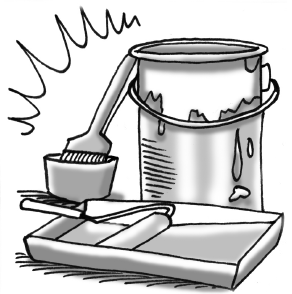### Home > INT2 > Chapter 11 > Lesson 11.2.3 > Problem11-83

11-83.Cindy’s cylindrical paint bucket has a diameter of 12 inches and a height of $14.5$ inches. If $1$ gallon $≈ 231\text{ in}^3$, then how many gallons does her paint bucket hold?

Volume of Cylinder = (area of base)(height) = (πr2)(h) = (36π in3)(14.5 in) ≈ 1640 in3

$\frac{1640\;\text{in}^{3}}{1}\cdot\frac{1\;\text{gallon}}{231\;\text{in}^{3}}=...$

$≈ 7.1$ gallons